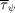International Tables for Crystallography (2019). Vol. H, ch. 5.4, pp. 581-600https://doi.org/10.1107/97809553602060000969

## Contents

• 5.4. Thin films and multilayers  (pp. 581-600)
• 5.4.1. Introduction  (p. 581) | html | pdf |
• 5.4.2. Effects of absorption  (p. 581) | html | pdf |
• 5.4.2.1. Absorption factor  (pp. 581-582) | html | pdf |
• 5.4.2.2. Chemical phase identification  (pp. 582-583) | html | pdf |
• 5.4.2.3. Determination and interpretation of the product μt  (pp. 583-584) | html | pdf |
• 5.4.2.4. Phase inhomogeneities  (p. 584) | html | pdf |
• 5.4.3. Grazing-incidence configurations  (pp. 584-590) | html | pdf |
• 5.4.3.1. Grazing-incidence X-ray diffraction (GIXRD)  (pp. 584-587) | html | pdf |
• 5.4.3.2. Penetration depth and information depth  (p. 587) | html | pdf |
• 5.4.3.3. Depth-dependent properties  (pp. 587-588) | html | pdf |
• 5.4.3.4. Index of refraction for X-rays  (p. 588) | html | pdf |
• 5.4.3.5. Total external reflection and critical angle  (pp. 588-590) | html | pdf |
• 5.4.3.6. Use of synchrotron radiation  (p. 590) | html | pdf |
• 5.4.4. Thin-film textures and their depth dependence  (pp. 590-593) | html | pdf |
• 5.4.4.1. Texture factors  (p. 590) | html | pdf |
• 5.4.4.2. Pole-figure scans and analysis  (pp. 590-592) | html | pdf |
• 5.4.4.3. Texture gradients  (pp. 592-593) | html | pdf |
• 5.4.5. Stress and strain analysis  (pp. 593-594) | html | pdf |
• 5.4.6. X-ray reflectivity (XRR)  (pp. 594-597) | html | pdf |
• 5.4.6.1. Reflectivity from a substrate  (pp. 594-595) | html | pdf |
• 5.4.6.2. Reflectivity of a single layer  (pp. 595-596) | html | pdf |
• 5.4.6.3. Reflectivity of multilayers and superlattices  (pp. 596-597) | html | pdf |
• 5.4.7. Grazing-incidence X-ray scattering (GIXS)  (pp. 597-598) | html | pdf |
• 5.4.8. Conclusions and perspective  (p. 598) | html | pdf |
• References | html | pdf |
• Figures
• Fig. 5.4.1. Schematic representation of absorption in a thin-film sample during a θ/2θ scan  (p. 581) | html | pdf |
• Fig. 5.4.2. Absorption factor A for 500 nm and 1 µm Al and Nb films as function of 2θ  (p. 582) | html | pdf |
• Fig. 5.4.3. Schematics of absorption in a multilayer system  (p. 583) | html | pdf |
• Fig. 5.4.4. Visualization of geometrical thickness (left) and projected thickness (right)  (p. 584) | html | pdf |
• Fig. 5.4.5. The geometry in grazing-incidence diffraction is characterized by a small incidence angle α that is kept constant during measurement  (p. 585) | html | pdf |
• Fig. 5.4.6. The two diffractometer configurations most frequently applied in GIXRD  (p. 585) | html | pdf |
• Fig. 5.4.7. Schematics of the illuminated sample depth for the derivation of the absorption factor Aα in GIXRD  (p. 586) | html | pdf |
• Fig. 5.4.8. The absorption factor Aα in GIXRD geometry for various incidence angles α and values of the product μt  (p. 586) | html | pdf |
• Fig. 5.4.9. (a) GIXRD, α = 1.8°, and (b) θ/2θ diffraction pattern from the same TiO2/Ti layer system on steel  (p. 587) | html | pdf |
• Fig. 5.4.11. Penetration depth τ1/e as function of incident angle α for TiO2 and Cu Kα radiation according to (5.4.45) (straight line) and (5.4.23) (dashed line)  (p. 589) | html | pdf |
• Fig. 5.4.12. Schematic drawing for deriving the Bragg peak position shift in GIXRD due to the refraction of the incoming beam  (p. 590) | html | pdf |
• Fig. 5.4.13. Average information depthas a function of tilt angle ψ for a 2θ = 32° reflection from thin BaTiO3 films  (p. 591) | html | pdf |
• Fig. 5.4.14. Thickness dependence of twist Δϕ for yttria-stabilized zirconia (YSZ) and Gd2Zr2O7 films  (p. 592) | html | pdf |
• Fig. 5.4.15. Resistivity of thin ZnO:Al films versus reciprocal texture index 1/J (Birkholz et al  (p. 592) | html | pdf |
• Fig. 5.4.16. Variation of the products μt of representative thin-film materials having high, average and low electron densities in the X-ray energy range between 5 and 15 keV  (p. 593) | html | pdf |
• Fig. 5.4.17. Simulation of an X-ray reflectivity scan from a quartz glass substrate of varying surface roughness σ probed by Cu Kα radiation  (p. 595) | html | pdf |
• Fig. 5.4.18. Scheme of reflected and refracted beams for deriving the phase difference Δ  (p. 595) | html | pdf |
• Fig. 5.4.19. Simulated X-ray reflectivity of a Ta2O5 thin film, t = 25 nm, on a fused silica substrate recorded with Cu Kα radiation  (p. 596) | html | pdf |
• Fig. 5.4.20. Notion of participating wavevectors of XRR of a multilayer system  (p. 596) | html | pdf |
• Fig. 5.4.21. Simulated X-ray reflectivity patterns of barrier coating SnO/NiCrOx/Ag/ZnO/SnO on glass recorded with Cu Kα radiation  (p. 597) | html | pdf |
• Fig. 5.4.22. Simulation of X-ray reflectivity of a (TiO2/C) superlattice on an Si wafer  (p. 597) | html | pdf |
• Tables
• Table 5.4.1. Mass densities ρ, according to Weast (1986), electron densities ρe and critical angles αc of some oxides used as optical coatings  (p. 588) | html | pdf |